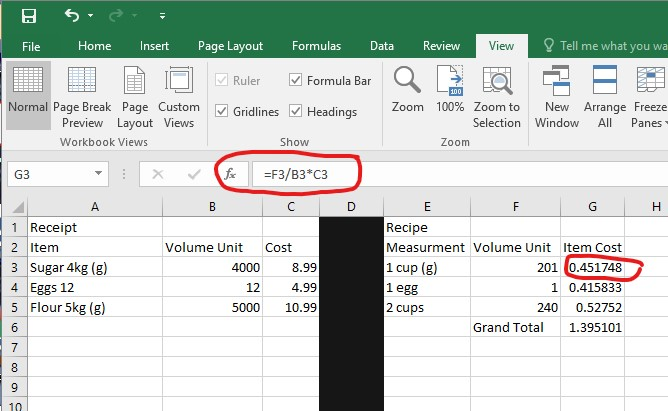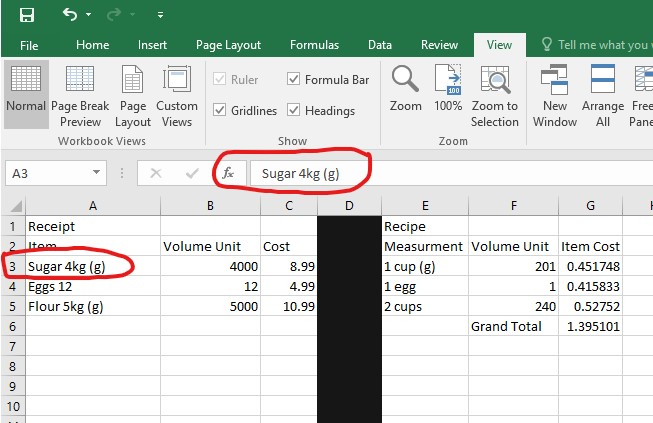# Project Cost Analysis

Overview:

This lesson plan is intended to help you integrate technology into your classroom. The use of the program Excel can enhance math and business practices. Excel is most likely new to your students, please familiarize yourself with this plan before teaching. Below is the pedagogical and contextual expectations as well as steps to follow to ensure the success of this lesson.

### Knowledge and Content

• understand the factors involved in assessing a product’s value and creating revenue
• comparing volumetric units
• converting units of measure
• understand the material value of a product
• assess whether or not their product is worth producing
• mathematical operations (multiplication, division, addition)
• define how much one must charge in order to make a profit

### Pedagogical Knowledge

• Identify a recipe
• purchase materials
• investigate volumes, weights, units of measure
• data entry
• copy and paste functions

### Technological Knowledge

This project could be done by hand on paper using basic math principals, however, this method is time consuming and does not align with the heavily digitized realty of the modern workplace. By using a pre-programed excel sheet, these same math functions will be automatically completed and the final product will perform faster and can be used repeatedly for future projects. The key knowledge in this lesson is not specifically mathematical but is specific to data entry, computer navigation and an introduction to excel.

#### Lesson Enhanced Through Technology

• Communication:
• Digitally presenting data
• Labeling files
• File sharing
• Accessing Content:
• Navigate USB key for specific file
• Opening the correct application (excel)
• Creating Content:
• Saving file using specific name/label
• Organizing Content:
• Save file to USB
• Create file copies

### SAMR

• Substitution
• Use the file as a digital receipt creator
• Augmentation
• Use the file to define a specific objective (cost of a single unit)
• use digital scale to compare volumetric weights
• Modification
• Use file to predict the cost of projects and material value
• use digital scale to compare volumetric weights
• Redefinition
• Use file to predict the cost of projects and material value,
• have student perform task in real time using a “google doc excel sheet”
• have students perform internet search to define volumetric weight conversions (1 cup sugar to grams)

### Technological Content and Knowledge

Throughout this lesson students will:

• Identify and open Excel
• Identify cells by columns and rows
• Enter data into specific cells
• Read and define cell functions
• Copy and paste functions (ctrl+C/ctrl+V)
• Print a copy of their file
• Save and store the digital file (Remind students to save multiple times)

#### Excel Formulas

• Begin a formula in any cell with an =
• =SUM specifies an addition formula
• =SUM(Cell:Cell) specifies the range that formula will cover
• =Cell*Cell specifies multiplication of a cell
• =Cell/Cell specifies division of a cell

### Technological Pedagogy and Knowledge:

It is important to have students follow the steps slowly, one at a time. Stop and check student’s status after each step depending on their experience and ability. (Teacher notes will be in brackets)

##### Materials:
• Receipt copies (either photo copy or multiple store receipts)
• A recipe and copies of whatever you are making
• USB key for each student
• Digital scale
• Bowls/plates
• measuring cups/spoons
• Student Laptops
##### Glossary of terms & Utilities:

Cell: A space on a grid or spreadsheet, specified with a column letter and a row number, example A7.

Cost: The amount of money something is worth.

Excel: Microsoft program designed for data entry

File explorer: Windows 10 system program to view files in folders

Formula Bar: the area that shows what formulas are in a cell

Start menu: Located in the bottom left corner or by using the windows key, this navigation tool allows you to explore the computers files, applications, internet search and more.

Volume: The measurement of space that a material occupies.

#### Steps:

##### 1. Insert the USB key

Demonstrate the proper method to insert and eject a USB key

##### 2. File Explorer

Open the file explorer by double clicking the file explorer icon or by finding it in the search bar.

##### 3. Navigate

Locate the USB key with the cost analysis file inside

Double click or Right click and open the file with excel

The file is preprogrammed and only requires data entry. It will look like the image below. The data already there is just an example.

##### 4. Make Observations

Before modifying any data, explore with the students, identify the important parts. (rows, columns, function bar, cell)

• Rows use numbers
• Columns use letters (like the game battleship)
• Click on ‘Sugar’ cell A3, students can type into the cell directly or into the function bar above
• Note that the text in the cell and the bar are the same
• Click on cell G3
• Note that the text in the cell and the bar are different• Note the difference between the numbers in the cell and the text in the formula bar. The cell shows a number but the bar shows =F3/B3*C3, this is a formula, a mathematical operation programmed into a cell.
• All formulas begin with an = which translates to this cell equals whatever follows. In this case, cell G3 equals cell F3 divided by cell B3 multiplied by cell C3. This is not a simple formula, but it can easily be copied and pasted.
• The formula shows the cost of the recipe compared to the original cost of the items
##### 5. Common unit of Measure

Before you start entering data, it is important to find a unit of measurement that is shared with the two different kinds of data. A common unit of measure is mandatory for the formula to function. This can be done by weighting the items, measuring with a measuring cup (fluids), or by researching on the internet. (how many grams of sugar are in a cup of sugar)

In the sample, sugar was purchased in kg, the recipe measured sugar by cup, the common unit of measure selected was grams. The solution, convert kg to g, and convert cup to g, this was through a google search.

##### 6. Data Entry (receipt)

Now that you’re familiar with the data formulas, it is time to start entering your own data.
Begin in cell A7 (below the sample data).

1. Enter the items from the original receipt,
• specify the name of the item
• the weight
• the common unit of measure
2. You and your students will have to come to an agreement on what the common measurement could be. Ask leading questions such as, is it a solid or a liquid, will we use ml, g, l, kg, lbs, oz?
3. Once you have a common unit of measure you can enter the value into column B (B7)
4. Finally enter the cost of the item.
• Note that food items do not usually have tax, but they also might benefit from the northern food subsidies.
##### 7. Data entry (recipe)

Beginning in cell E7,

1. Enter the unit of measurement for each item specified by the recipe
• specify the common unit of measurement
2. In column F enter the value of the common unit of measure.
• This number can be found by weighing, measuring or performing an internet search.
3. Repeat the steps for data entry for each item until finished
##### 8. Copy and Paste Forumlas

All the data should be entered in the cells except for column G.

Select cell G3, which contains the formula, right click and copy,

Paste into G7, hit enter. The cells used in the formula will automatically update themselves to respond to the row you are using.

The cell should now show a number that equals the portion of money the recipe actually uses.

Repeat the paste action for each item until finished.

##### 9. Grand Total

At the bottom of your items in column F students can type Grand Total.

To calculate the grand total, simply copy the formula from cell G6 and paste at the end of column G, then specify in the formula which cells will be added together by dragging the coloured rectangle over the area you wish to add or by typing the range of cells (G7:G16).

##### 10. Using the data

Now that the cost of your recipe/project has been isolated this information can be used to

• Plan future activities
• Setting an appropriate sale price for the product
• Plan how many recipes can be created with the remaining supplies
• Identifying margins of error and loss
• Identifying major expenses